# Highlight Row with Bottom 3 Values with a Criteria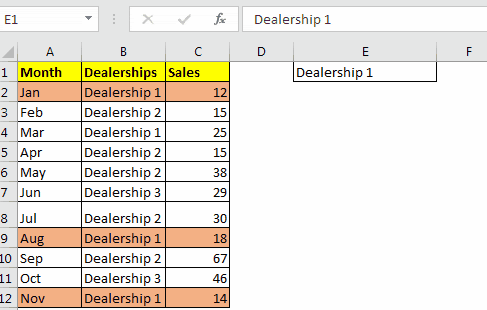We highlight cells in excel so that we can easily identify the focus areas on data. Formatting of cells in excel is also done for visualising data values based on colour and style. For example, you may want to focus on the 3 smallest values in your data. It's hard to do in a large data but if you could tell excel to highlight 3 lowest values then it would be quite easy. Fortunately, we can do that using conditional formatting option in Excel.

It is easy to just highlight 3 smallest value in excel but it gets a little bit tricky when we add criteria. In this article, we will learn how to highlight the smallest 3 values with a condition.

Generic Formula in Conditional Formating

=AND(check cell=criteria, value cell<=SMALL(IF(criteria range=criteria,value range),3))

Check cell: It is the cell that you want to check if it meats the first criteria (the category).

Criteria: The criteria that you want to filter.

Value cell: It is the cell that contains a numeric value. We will compare it with 3rd smallest value in its category, in the value range.

Criteria range: It is the absolute range that contains the criteria.

Value range: The range that contains all values.

Let’s see an example to make things clear.
Example: Highlight the bottom three sales done by Department 1
Here I have a sample work file that contains sales done by different departments in different months.

I want to highlight the bottom three sales done by Department 1.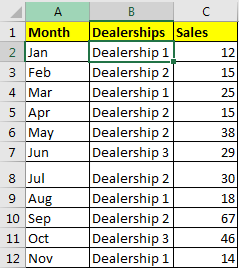Let's identify the variables we have here.

Check cell: \$B2 (we want to check if B2 contains "dealership 1". Since we don't want the column to change, we use \$ sign. This makes the whole row highlight.)

Criteria: "Department 1"

Value cell: \$C2

Criteria range: \$B\$2:\$B\$12

Value range: \$C\$2:\$C\$12

Now that we know all the arguments we need to pass to the formula, its time to implement the above generic formula on data set.

• Select the data. Go-to home ? conditional formatting ? New Rule
•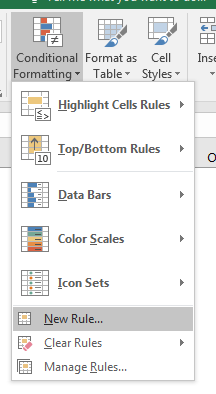• Select "Use a formula to determine which cell to format" option.
•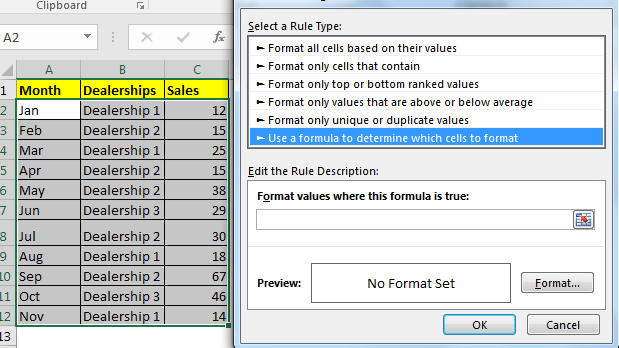• In the text box below, write this formula
• =AND(\$B2="Dealership 1",\$C2<=SMALL(IF(\$B\$2:\$B\$12="Dealership 1",\$C\$2:\$C\$12),3))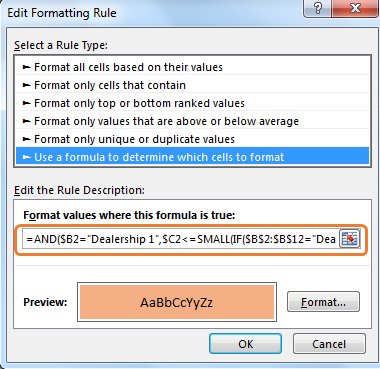• Select the formatting from using format button. I have used orange (maybe, don't judge my gender) fill.

Hit the OK button. And it's done. Bottom three or say smallest 3 sales from department 1 is highlighted.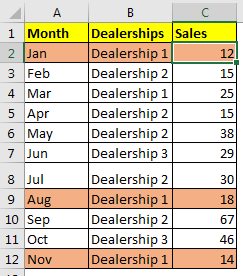How does it work?

Let's break down the formula from inside.

This formula is an array formula but CTRL+SHIFT+ENTER is not needed in conditional formatting.

Since we have two conditions, first is the Dealership 1 and second is values less than or equal to 3rd smallest value in Dealership 1. Both need to be TRUE. For that, we use AND function.

\$B2="Dealership 1"

First criteria is easy to check. We simply put this statement \$B2="Dealership 1". It will be TRUE if the current cell B2 contains Dealership 1.

\$C2<=SMALL(IF(\$B\$2:\$B\$12="Dealership 1",\$C\$2:\$C\$12),3)

For the second criteria, we need to filter Dealership 1 and find the 3rd smallest value in it. Then compare the criteria cell with 3rd smallest value. This is done using the statement \$C2<=SMALL(IF(\$B\$2:\$B\$12="Dealership 1",\$C\$2:\$C\$12),3)

When we evaluate it, this formula will solve in this way:

We check range \$B\$2:\$B\$12 and get cells that contain "Dealership 1" using statement \$B\$2:\$B\$12="Dealership 1". We will have an array of TRUE and FALSE.

\$C2<=SMALL(IF({TRUE;FALSE;TRUE;FALSE;FALSE;FALSE;FALSE;TRUE;FALSE;FALSE;TRUE},\$C\$2:\$C\$12),3)

For each TRUE, value will be returned from value range \$C\$2:\$C\$12 and we will have another array.

\$C2<=SMALL({12;FALSE;25;FALSE;FALSE;FALSE;FALSE;18;FALSE;FALSE;14},3)

Now SMALL Function will return the 3rd smallest value from this array. (FLASE will be ignored).

Now we have:

\$C2<=18

Finally, the formula is

=AND(\$B2="Dealership 1",\$C2<=18)

Now it checks If B2 contains Dealership 1. It does. Hence it is TRUE

=AND(TRUE,\$C2<=18)

Next, it checks if C2 is less then or equal to 18 (third smallest sales in dealership 1). It is TRUE (12).

=AND(TRUE, TRUE)

Since both statements are TRUE the formula returns TRUE and we get our first ROW highlighted with colour. Same happens in each row.

Fewww!!! It was tough to explain. I hope I was clear enough to make you understand the method. In practice, it is easy.

Here we used hardcoded criteria but we can also give cell reference too. This will make the formula more flexible.

Here, for Criteria I have used cell reference of E1. Whichever dealership you have in E1, the three smallest value from that dealership will be highlighted.The formula in conditional formatting is:

=AND(\$B2=\$E\$1,\$C2<=SMALL(IF(\$B\$2:\$B\$12=\$E\$1,\$C\$2:\$C\$12),3))

So yeah, this how you can highlight bottom three value with a condition in excel. I hope the article was helpful. If you have any query, please use the comments section below. You can ask questions related to this article or any other excel related article. We will be happy to help you.

Related Articles:

Conditional formatting based on another cell value

IF and Conditional formatting

Conditional Formatting with formula 2016

Conditional Formatting using VBA in Microsoft Excel

Highlight cells that contain specific text

How to Sum Multiple Columns with Condition

Popular Articles:

50 Excel Shortcut’s to Increase Your Productivity

The VLOOKUP Function in Excel

COUNTIF in Excel 2016

How to Use SUMIF Function in Excel

Terms and Conditions of use

The applications/code on this site are distributed as is and without warranties or liability. In no event shall the owner of the copyrights, or the authors of the applications/code be liable for any loss of profit, any problems or any damage resulting from the use or evaluation of the applications/code.